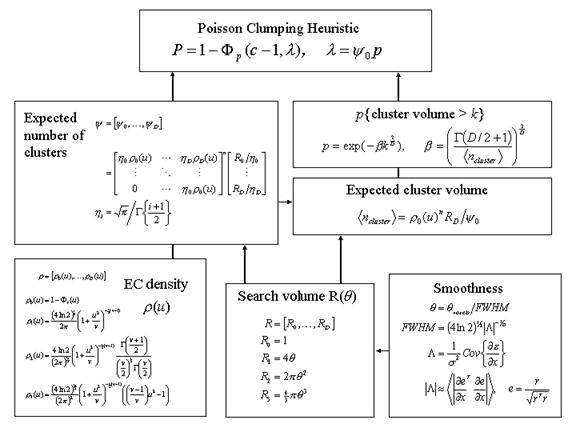Figure 7

Schematic illustrating the use of Random Field theory in making inferences about SPMs. If one knew where to look exactly, then inference can be based on the value of the statistic at a specified location in the SPM, without correction. However, if one did not have an anatomical constraint a priori, then an adjustment for multiple dependent comparisons has to be made. These corrections are usually made using distributional approximations from GRF theory. This schematic deals with a general case of n SPM{T} whose voxels all survive a common threshold u (i.e. a conjunction of n component SPMs). The central probability, upon which all voxel, cluster or set-level inferences are made, is the probability P of getting c or more clusters with k or more resels (resolution elements) above this threshold. By assuming that clusters behave like a multidimensional Poisson point process (i.e. the Poisson clumping heuristic) P is simply determined. The distribution of c is Poisson with an expectation that corresponds to the product of the expected number of clusters, of any size, and the probability that any cluster will be bigger than k resels. The latter probability is shown using a form for a single Z-variate field constrained by the expected number of resels per cluster (<.> denotes expectation or average). The expected number of resels per cluster is simply the expected number of resels in total divided by the expected number of clusters. The expected number of clusters is estimated with the Euler characteristic (EC) (effectively the number of blobs minus the number of holes). This estimate is in turn a function of the EC density for the statistic in question (withdegrees of freedom v) and the resel counts. The EC density is the expected EC per unit of D-dimensional volume of the SPM where the D dimensional volume of the search space is given by the corresponding element in the vector of resel counts. Resel counts can be thought of as a volume metric that has been normalized by the smoothness of the SPMs component fields expressed in terms of the full width at half maximum (FWHM). This is estimated from the determinant of the variance-covariance matrix of the first spatial derivatives of e, the normalized residual fields r (from Figure 4.htm). In this example equations for a sphere of radius q are given. F denotes the cumulative density function for the sub-scripted statistic in question.